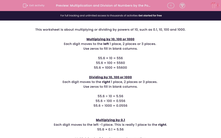# Multiplication and Division of Numbers by the Powers of 10 (0.1 - 1000)

In this worksheet, students must multiply or divide numbers by powers of 10 (0.1 to 1000).This content is premium and exclusive to EdPlace subscribers.Key stage:  KS 2

Curriculum topic:   Maths and Numerical Reasoning

Curriculum subtopic:   Mixed Problems

Difficulty level:#### Worksheet Overview

This worksheet is about multiplying or dividing by powers of 10, such as 0.1, 10, 100 and 1000.

Multiplying by 10, 100 or 1000

Each digit moves to the left 1 place, 2 places or 3 places.

Use zeros to fill in blank columns.

55.6 × 10 = 556

55.6 × 100 = 5560

55.6 × 1000 = 55600

Dividing by 10, 100 or 1000

Each digit moves to the right 1 place, 2 places or 3 places.

Use zeros to fill in blank columns.

55.6 ÷ 10 = 5.56

55.6 ÷ 100 = 0.556

55.6 ÷ 1000 = 0.0556

Multiplying by 0.1

Each digit moves to the left -1 place. This is really 1 place to the right.

55.6 × 0.1 = 5.56

Multiplying by 0.1 makes a number smaller.

Dividing by 0.1

Each digit moves to the right -1 place. This is really 1 place to the left.

55.6 ÷ 0.1 = 556

Dividing by 0.1 makes a number bigger.

### What is EdPlace?

We're your National Curriculum aligned online education content provider helping each child succeed in English, maths and science from year 1 to GCSE. With an EdPlace account you’ll be able to track and measure progress, helping each child achieve their best. We build confidence and attainment by personalising each child’s learning at a level that suits them.

Get started